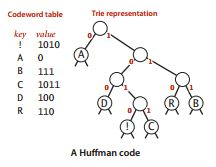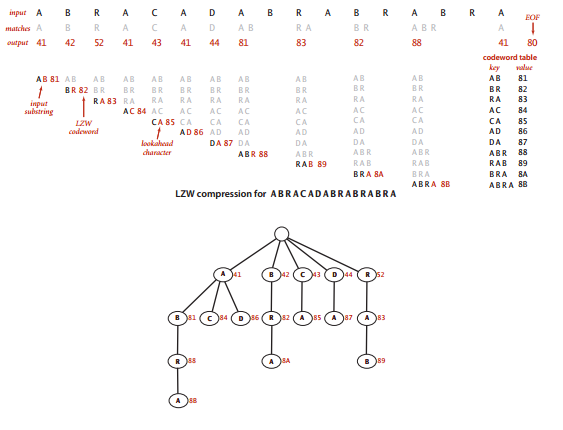# 《算法》笔记 17 - 数据压缩

• 读写二进制数据
• 基因组数据的压缩
• 游程编码
• 位图
• 霍夫曼压缩
• 前缀码和单词查找树
• 构造前缀码的单词查找树
• 写入和读取单词查找树
• 使用前缀码压缩
• 使用前缀码展开
• LZW压缩
• LZW的单词查找树
• LZW的单词查找树
• LZW压缩的展开

### 基因组数据的压缩

public static void compress(){
Alphabet DNA=new Alphabet("ACTG");
int N=s.length();
BinaryStdOut.write(N);

for(int i=0;i<N;i++){
int d=DNA.toIndex(s.charAt(i));
BinaryStdOut.write(d,DNA.lgR());
}
BinaryStdOut.close();
}


public static void expand(){
Alphabet DNA=new Alphabet("ACTG");
int w=DNA.lgR();
for(int i=0;i<N;i++){
StdOut.println("c="+c);
char e=DNA.toChar(c);
StdOut.println("e="+e);
BinaryStdOut.write(DNA.toChar(c));
}
BinaryStdOut.close();
}


### 游程编码

#### 位图

00000000000000000000000000000000
00000000000000000000000000000000
00000000000000011111110000000000
00000000000011111111111111100000
00000000001111000011111111100000
00000000111100000000011111100000
00000001110000000000001111100000
00000011110000000000001111100000
00000111100000000000001111100000
00001111000000000000001111100000
00001111000000000000001111100000
00011110000000000000001111100000
00011110000000000000001111100000
00111110000000000000001111100000
00111110000000000000001111100000
00111110000000000000001111100000
00111110000000000000001111100000
00111110000000000000001111100000
00111110000000000000001111100000
00111110000000000000001111100000
00111110000000000000001111100000
00111111000000000000001111100000
00111111000000000000001111100000
00011111100000000000001111100000
00011111100000000000001111100000
00001111110000000000001111100000
00001111111000000000001111100000
00000111111100000000001111100000
00000011111111000000011111100000
00000001111111111111111111100000
00000000011111111111001111100000
00000000000011111000001111100000
00000000000000000000001111100000
00000000000000000000001111100000
00000000000000000000001111100000
00000000000000000000001111100000
00000000000000000000001111100000
00000000000000000000001111100000
00000000000000000000001111100000
00000000000000000000001111100000
00000000000000000000001111100000
00000000000000000000001111100000
00000000000000000000001111100000
00000000000000000000011111110000
00000000000000000011111111111100
00000000000000000111111111111110
00000000000000000000000000000000
00000000000000000000000000000000


00000000001111000011111111100000


4位编码能表示的值只有0到15，在实际的应用中是不够的，所以使用8位编码，可以表示0-255之间的游程长度。如果游程的长度超过256，就插入一个长度为0的游程，这样可以确保所有的游程长度都不超过256。

public static void compress() {
char cnt = 0;
boolean b, old = false;
while (!BinaryStdIn.isEmpty()) {
if (b != old) {
BinaryStdOut.write(cnt);
cnt = 0;
old = !old;
} else {
if (cnt == 255) {
BinaryStdOut.write(cnt);
cnt = 0;
BinaryStdOut.write(cnt);
}
}
cnt++;
}
BinaryStdOut.write(cnt);
BinaryStdOut.close();
}


public static void expand() {
boolean b = false;
while (!BinaryStdIn.isEmpty()) {
for (int i = 0; i < cnt; i++)
BinaryStdOut.write(b);
b = !b;
}
BinaryStdOut.close();
}


### 霍夫曼压缩

#### 前缀码和单词查找树

A:0
B:1
R:00
C:01
D:10• 压缩时：
• 构造前缀码的单词查找树
• 将树以字节流的形式输出
• 使用构造的树将字节流编码为比特流
• 展开时：
• 读取单词查找树
• 使用该树将比特流解码

#### 构造前缀码的单词查找树

##### 单词查找树的结点
public static class Node implements Comparable<Node> {
private char ch;
private int freq;
private final Node left, right;

Node(char ch, int freq, Node left, Node right) {
this.ch = ch;
this.freq = freq;
this.left = left;
this.right = right;
}

public boolean isLeaf() {
return left == null && right == null;
}

public int compareTo(Node that) {
return this.freq - that.freq;
}
}


##### 构造单词查找树

private static Node buildTrie(int[] freq) {
MinPQ<Node> pq = new MinPQ<Node>();
for (char c = 0; c < R; c++)
if (freq[c] > 0)
pq.insert(new Node(c, freq[c], null, null));
while (pq.size() > 1) {
Node x = pq.delMin();
Node y = pq.delMin();
Node parent = new Node('\0', x.freq + y.freq, x, y);
pq.insert(parent);
}
return pq.delMin();
}


#### 写入和读取单词查找树

##### 写入

private static void writeTrie(Node x) {
if (x.isLeaf()) {
BinaryStdOut.write(true);
BinaryStdOut.write(x.ch);
return;
}
BinaryStdOut.write(false);
writeTrie(x.left);
writeTrie(x.right);
}

##### 读取

private static Node readTrie() {
return new Node(BinaryStdIn.readChar(), 0, null, null);
}


#### 使用前缀码压缩

private static void buildCode(String[] st, Node x, String s) {
if (x.isLeaf()) {
st[x.ch] = s;
return;
}
buildCode(st, x.left, s + '0');
buildCode(st, x.right, s + '1');
}


public static void compress() {
char[] input = s.toCharArray();

int[] freq = new int[R];
for (int i = 0; i < input.length; i++)
freq[input[i]]++;

Node root = buildTrie(freq);
String[] st = new String[R];
buildCode(st, root, "");
writeTrie(root);
BinaryStdOut.write(input.length);
for (int i = 0; i < input.length; i++) {
String code = st[input[i]];
for (int j = 0; j < code.length(); j++)
if (code.charAt(j) == '1')
BinaryStdOut.write(true);
else
BinaryStdOut.write(false);
}
BinaryStdOut.close();
}


#### 使用前缀码展开

public static void expand() {
for (int i = 0; i < N; i++) {
Node x = root;
while (!x.isLeaf())
x = x.right;
else
x = x.left;
BinaryStdOut.write(x.ch);
}
BinaryStdOut.close();
}


### LZW压缩

LZW算法的基本思想和霍夫曼压缩的思想正好相反。霍夫曼压缩是为输入中的定长模式产生了变长的编码编译表；而LZW压缩是为输入中的变长模式生成了一张定长的编码编译表。而且，LZW算法不需要在输出中附上这张编译表。

LZW压缩算法的基础是维护一张字符串键和编码的编译表。在符号表中，将128个ASCII码的值初始化为8位编码，即在每个字符的编码值前面添加0。然后用16进制数字来表示编码，那么A的编码就是41，R的是52等等。将80编码保留为文件借宿的标志，并将81-FF的编码值分配给在输入中遇到的各种子字符串。

• 找出未处理的输入在符号表中最长的前缀字符串s；
• 输出s的8位编码值；
• 继续扫描s之后的一个字符c；
• 在符号表中将s+c（连接s和c）的值设为下一个编码值。#### LZW的单词查找树

LZW算法会用到三向单词查找树，包含两种操作：

• 找到输入和符号表的所有键的最长前缀匹配；
• 将匹配的键和前瞻字符相连得到一个新键，将新键和下一个编码管理并添加到符号表中。

#### LZW压缩的展开

• 输出当前字符串val；
• 从输入中读取一个编码x；
• 在符号表中将s设为和x相关联的值；
• 在符号表中将下一个未分配的编码值设为val+c，其中c为s的首字母；
• 将当前字符串val设为s；

public class LZW {
private static final int R = 256;
private static final int L = 4096;
private static final int W = 12;

public static void compress() {
TST<Integer> st = new TST<Integer>();

for (int i = 0; i < R; i++)
st.put("" + (char) i, i);
int code = R + 1;

while (input.length() > 0) {
String s = st.longestPrefixOf(input);
BinaryStdOut.write(st.get(s), W);

int t = s.length();
if (t < input.length() && code < L)
st.put(input.substring(0, t + 1), code++);
input = input.substring(t);
}

BinaryStdOut.write(R, W);
BinaryStdOut.close();
}

public static void expand() {
String[] st = new String[L];
int i;
for (i = 0; i < R; i++)
st[i] = "" + (char) i;
st[i++] = " ";

String val = st[codeword];
while (true) {
BinaryStdOut.write(val);
if (codeword == R)
break;
String s = st[codeword];
if (i == codeword)
s = val + val.charAt(0);
if (i < L)
st[i++] = val + s.charAt(0);
val = s;
}
BinaryStdOut.close();
}
}

posted @ 2020-01-26 08:49  zhixin9001  阅读(...)  评论(...编辑  收藏